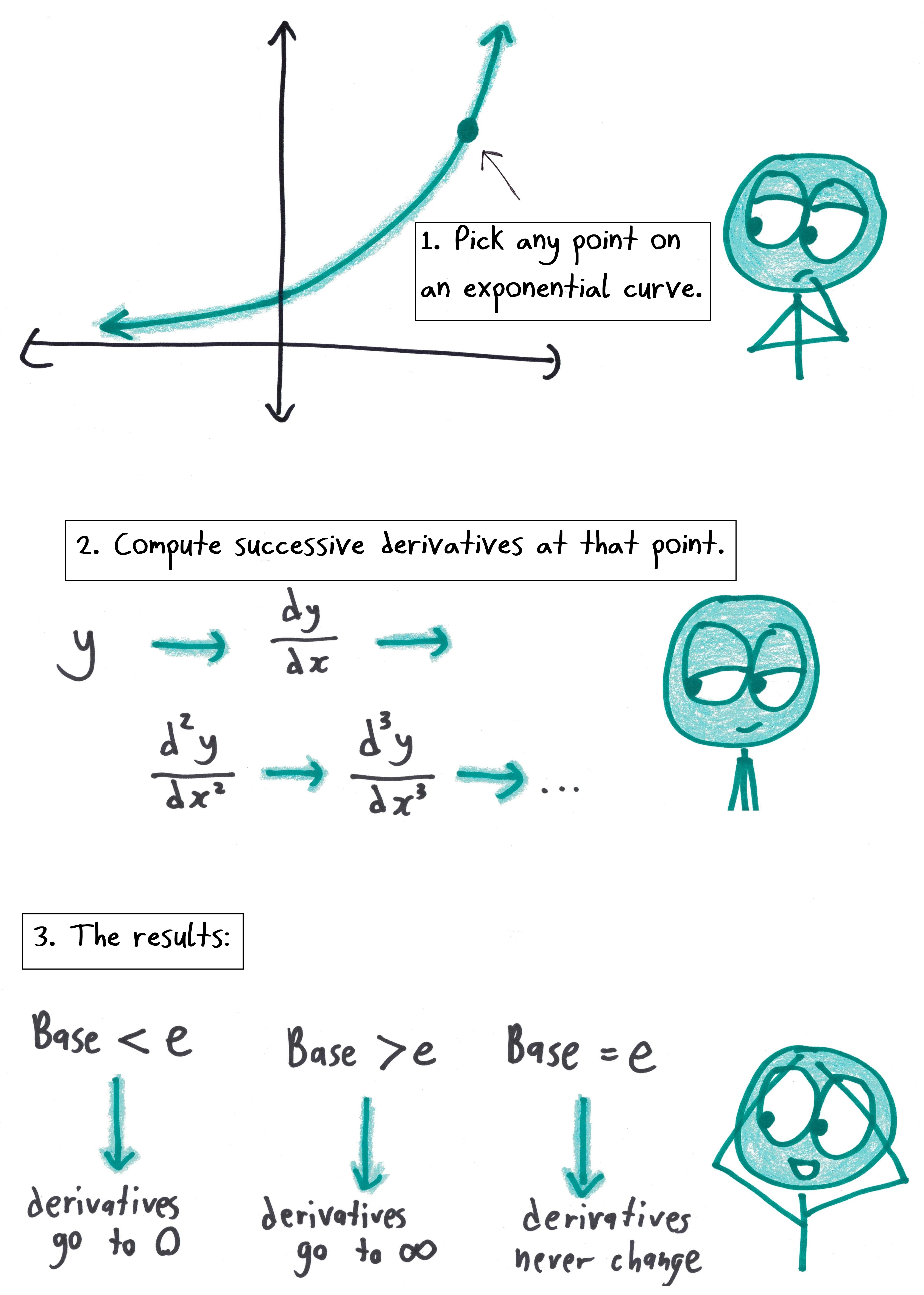# Truly the Natural Base

NOTE: Daily cartoons are now available only through Patreon. Just \$1 a month!

In the Homeric epic that is mathematics, gets a choice epithet. It is “the natural base.”

inventoried the reasons last year (for the historic once-in-a-century e Day). And I later stumbled across an even more delicious “naturalness” argument for e.

But that’s hardly exhaustive. Here’s another thing I dig about e, an easy fact for any first-year calculus student to verify:Also, an addendum: a fun observation from Twitter…

Addendum #2, on March 4, 2019: the inimitable Sam Shah asks how we can introduce e to algebra students, and then delivers a delightful post rounding up more lovely e facts.

## 6 thoughts on “Truly the Natural Base”

1.Jerry says:

Doesn’t Coya’s law hold for any logarithm, not just log base e? (except the unit law has e replaced by the base of the log.)

1.Ben Orlin says:

Good point!

2.(Mr) Bill says:

Thank you. Here are two more properties that make e unique among the reals. (Does unique ==> natural? Discuss among yourselves.)

(1) e is the positive real number with the greatest “self-root.” When posed as the problem, “Determine the maximum value of f(x) = x^(1/x),” this fact is the correct answer to a standard Calculus I exercise.
(2) The second fact is closely related to your yummy “naturalness” justification. Call two positive real numbers a and b _exponential B.F.F.s_ iff a ≠ b and a^b = b^a. Then e is the unique real number > 1 without an exponential B.F.F.

3.chridd says:

x#y is an operation I discovered on my own back when I was taking a class on ring/field theory. Some other properties:

1#x = 1^ln x = 1; x#1 = x^(ln 1) = x^0 = 1; so 1 behaves like 0 does in multiplication.

x#(e^(1/ln x)) = e, meaning e^(1/ln x) is the inverse of x (though it’s undefined if x = 1).

(xy)#z = (xy)^ln z = (x^ln z)(y^ln z) = (x#z)(y#z); that is, # distributes over multiplication just like multiplication distributes over addition.

The positive real numbers with the operations × and # are a field, and that field is isomorphic to the real numbers with the usual addition and multiplication.

4.howardat58 says:

For any x the tangent at (x, e^x) cuts the x axis at x – 1

5.mathdads says:

This law has been known for a while. A quick search provides a result 4 years old and I would guess a more thorough search would find one much, much older.